## Tuesday, May 31, 2016

### More on `plot` method for object class `"fitMk"`

I've just been playing with the S3 `plot` method that I added to phytools. Here's how it can be used for characters with two, three, four, five, or more states. I'm going to use simulated data just for illustrative purposes, except for one Anolis example at the very end:

``````library(phytools)
## two states
tree<-pbtree(n=26,tip.label=letters,scale=1)
Q<-matrix(c(-2,2,1,-1),2,2)
rownames(Q)<-colnames(Q)<-c("aquatic","terrestrial")
x<-as.factor(sim.history(tree,Q)\$states)
``````
``````## Note - the rate of substitution from i->j should be given by Q[j,i].
## Done simulation(s).
``````
``````fit2<-fitMk(tree,x,model="ARD")
plot(fit)
````````````## three states
tree<-pbtree(n=100,scale=1)
Q<-matrix(c(-1,1,0,0,-1,1,0,1e-12,-1e-12),3,3)
rownames(Q)<-colnames(Q)<-paste("state",c("I","II","III"))
x<-as.factor(sim.history(tree,Q,anc="state I")\$states)
``````
``````## Note - the rate of substitution from i->j should be given by Q[j,i].
## Done simulation(s).
``````
``````fit3<-fitMk(tree,x,model="ARD")
fit3
``````
``````## Object of class "fitMk".
##
## Fitted (or set) value of Q:
##             state I  state II state III
## state I   -0.631994  0.382593  0.249401
## state II   0.000000 -0.731563  0.731563
## state III  0.000000  0.000000  0.000000
##
## Fitted (or set) value of pi:
##   state I  state II state III
## 0.3333333 0.3333333 0.3333333
##
## Log-likelihood: -38.374895
``````
``````plot(fit3,cex.traits=0.8,cex.rates=0.8,mar=rep(0,4))
``````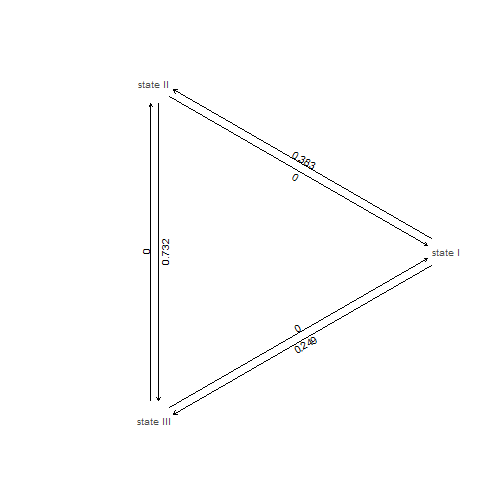``````plot(fit3,cex.traits=0.8,cex.rates=0.8,mar=rep(0,4),
show.zeros=FALSE)
``````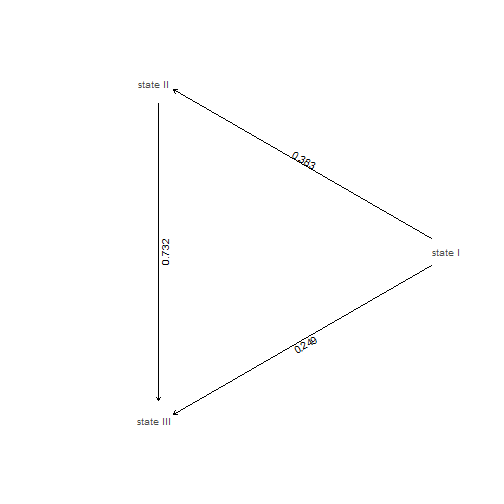``````## four states
tree<-pbtree(n=200,scale=1)
Q<-matrix(c(-1,1,0,0,
0,-1,1,0,
0,0,-1,1,
1,0,0,-1),4,4)
rownames(Q)<-colnames(Q)<-letters[1:4]
x<-as.factor(sim.history(tree,Q)\$states)
``````
``````## Note - the rate of substitution from i->j should be given by Q[j,i].
## Done simulation(s).
``````
``````fit4<-fitMk(tree,x,model="ARD")
plot(fit4,show.zeros=FALSE)
``````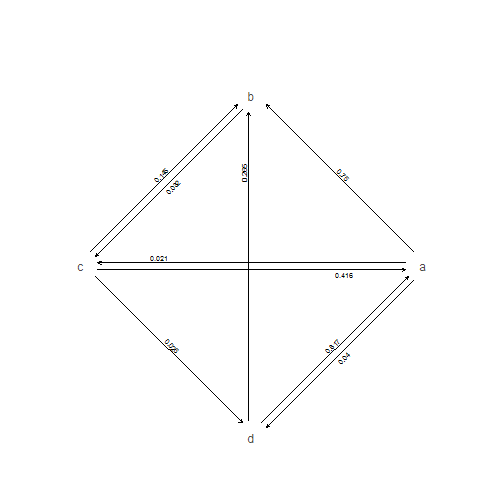``````## five states
Q<-matrix(c(0,3,2,1,0,
3,0,3,2,1,
2,3,0,3,2,
1,2,3,0,3,
0,1,2,3,0),5,5)
diag(Q)<--colSums(Q)
rownames(Q)<-colnames(Q)<-levels<-
c("some","slightly","longer","named","states")
x<-factor(sim.history(tree,Q)\$states,levels=levels)
``````
``````## Done simulation(s).
``````
``````fit5<-fitMk(tree,x,model="SYM")
plot(fit5,cex.traits=0.8)
``````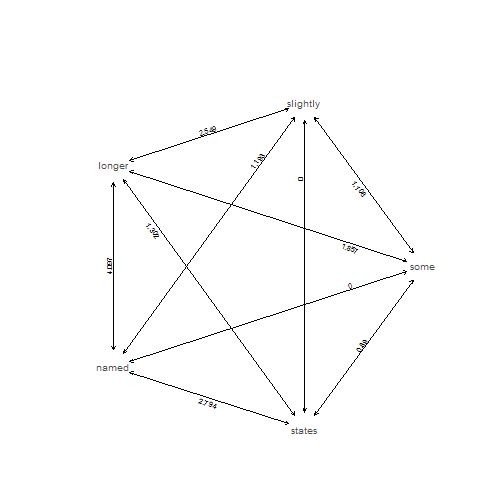``````## ten states
Q<-matrix(rep(1,100),10,10)
diag(Q)<-0
diag(Q)<--colSums(Q)
rownames(Q)<-colnames(Q)<-letters[1:10]
x<-sim.history(tree,Q)\$states
``````
``````## Done simulation(s).
``````
``````fitER<-fitMk(tree,x,model="ER")
plot(fitER)
``````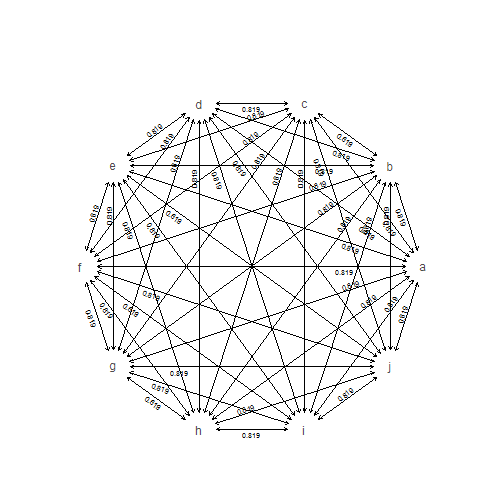Finally, let's load the Anolis data:

``````data(anoletree)
anoletree
``````
``````##
## Phylogenetic tree with 82 tips and 81 internal nodes.
##
## Tip labels:
##  Anolis_ahli, Anolis_allogus, Anolis_rubribarbus, Anolis_imias, Anolis_sagrei, Anolis_bremeri, ...
##
## The tree includes a mapped, 6-state discrete character with states:
##  CG, GB, TC, TG, Tr, Tw
##
## Rooted; includes branch lengths.
``````
``````x<-getStates(anoletree,"tips")
fitARD<-fitMk(anoletree,x,model="ARD")
``````
``````## Warning in log(comp[1:M + N]): NaNs produced

## Warning in log(comp[1:M + N]): NaNs produced

## Warning in log(comp[1:M + N]): NaNs produced

## Warning in log(comp[1:M + N]): NaNs produced

## Warning in log(comp[1:M + N]): NaNs produced

## Warning in log(comp[1:M + N]): NaNs produced

## Warning in log(comp[1:M + N]): NaNs produced

## Warning in log(comp[1:M + N]): NaNs produced

## Warning in log(comp[1:M + N]): NaNs produced

## Warning in log(comp[1:M + N]): NaNs produced

## Warning in log(comp[1:M + N]): NaNs produced

## Warning in log(comp[1:M + N]): NaNs produced

## Warning in log(comp[1:M + N]): NaNs produced

## Warning in log(comp[1:M + N]): NaNs produced

## Warning in log(comp[1:M + N]): NaNs produced

## Warning in log(comp[1:M + N]): NaNs produced

## Warning in log(comp[1:M + N]): NaNs produced

## Warning in log(comp[1:M + N]): NaNs produced

## Warning in log(comp[1:M + N]): NaNs produced

## Warning in log(comp[1:M + N]): NaNs produced

## Warning in log(comp[1:M + N]): NaNs produced

## Warning in log(comp[1:M + N]): NaNs produced

## Warning in log(comp[1:M + N]): NaNs produced

## Warning in log(comp[1:M + N]): NaNs produced

## Warning in log(comp[1:M + N]): NaNs produced

## Warning in log(comp[1:M + N]): NaNs produced
``````
``````plot(fitARD,show.zeros=FALSE,tol=1e-3,signif=4)
``````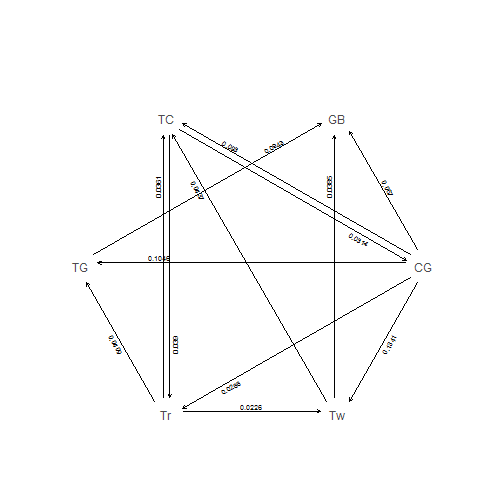(Note that I wouldn't put too much credence in this particular result. This is just way too many parameters to estimate from a tree with 82 tips!)

That's it.

1.This comment has been removed by the author.

2.Phylogenetic trees are central to the field of phylogenetics. I would love to see more written explanation of this field as it caught my interest. Would you have a second like to look into this, this useful review of grab my essay will surely interest you and will be a help for all of you.

3.How shall we deal with "Warning in log(comp[1:M + N]): NaNs produced"?

Note: due to the very large amount of spam, all comments are now automatically submitted for moderation.